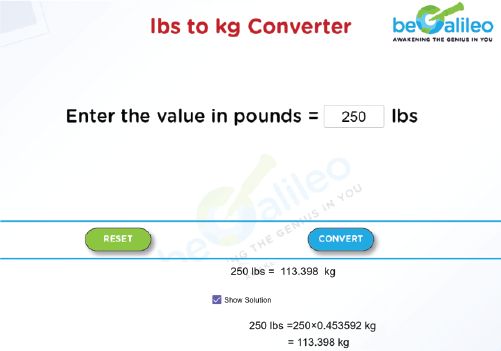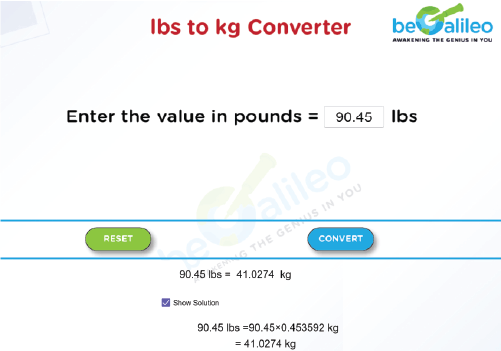>### Master Math with 1:1 Online Classes. Learn from Experts!## lbs TO kg CONVERTER

A lbs to kg Converter is a simple tool that plays a crucial role in our daily lives, particularly for those of us who find ourselves toggling between different measurement systems. Whether you're a fitness enthusiast tracking your weight in kilograms or planning a trip to a country that uses the metric system, this conversion tool is an essential companion.

The need for a pounds (lbs) to kilograms (kg) converter arises due to the coexistence of two distinct systems of measurement used across the globe. While the United States primarily relies on pounds to measure weight, many countries, including most of Europe, use kilograms.

## How to use a lbs to kg Converter?

Here's a step-by-step explanation of how a lbs to kg Converter works:

1. Input the Weight in Pounds :
Enter the weight in pounds you wish to convert.
2. Click on “Convert” :
After entering the weight in pounds, click the "Convert" button. This will start the conversion process.
3. View the Result :
The converted weight in kilograms will be displayed below the Converter. This is the equivalent weight in kg for the pounds you entered.
4. Reset Option :
If needed, you can use the reset button to clear all entered numbers, allowing you to correct input errors or start a new calculation.
If you want to view the calculation then click on the ‘Show Solution’ checkbox.

## How to convert lbs to kg?

To convert pounds (lbs) to kilograms (kg), you can use the following formula:

Weight in kilograms (kg) = Weight in pounds (lbs)\times0.453592

Simply multiply the weight in pounds by 0.453592 to obtain the equivalent weight in kilograms.

Example : Convert 150 lbs to kg.

Solution : Weight in pounds (lbs): 150

To convert this to kilograms, use the formula:

Weight in kilograms (kg) = Weight in pounds (lbs) × 0.453592

= 150 lbs\times0.453592 = 68.0388 kg

So, 150 pounds is equal to 68.0388 kilograms.

## Solved Examples

### Q1. Convert 250 pounds to kilograms.

Solution :
Weight in pounds (lbs): 250
Weight in kilograms (kg) = 250 lbs\times0.453592 = 113.398 kg
So, 250 pounds is equal to 113.398 kilograms.### Q2.Convert 90.45 pounds to kilograms.

Solution :
Weight in pounds (lbs): 90.45
Weight in kilograms (kg) = 90.45 Lbs\times0.453592 = 41.0274 kg
So, 90.45 pounds is equal to 41.0274 kilograms.